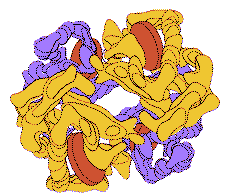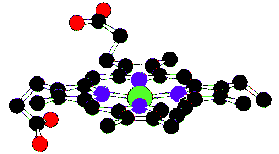#### Equivalents

One of the most confusing things about looking at hemoglobin (Hb) spectra is that the values are typically tabulated in equivalents. The term equivalent is used to indicate the amount of hemoglobin which contains 1 gm atom of Fe and combines with 1 gm molecule of O2 or CO. One equivalent of hemoglobin is assumed to be 64,500/4 or 16,125 gm. A concentration of 10-6 equivalent is 16.125mg of hemoglobin per cc.

Thus there are four times as many equivalents as there are hemoglobin molecules.

Despite the fact that others use equivalents, I will present the oxy and deoxy-hemoglobin spectra in terms of molar extinction coefficient. To convert from the molar extinction coefficient e to absorbance A, multiply by the molar concentration and the pathlength. For example, if x is the number of grams per liter and a 1 cm cuvette is being used, then the absorbance is given by

```        (e) [(1/cm)/(moles/liter)] (x) [g/liter] (1) [cm]
A =  ---------------------------------------------------
64,500 [g/mole]
```
using 64,500 as the gram molecular weight of hemoglobin.

#### Binding oxygen changes the spectra

If the hemoglobin molecule is bound to oxygen then one has oxy-hemoglobin or Hb02. If the hemoglobin molecule is bound to carbon monoxide then one has carboxy-hemoglobin or HbCO. If the hemoglobin molecule is bound to nothing then one has deoxy-hemoglobin or Hb. If the hemoglobin molecule has broken down then one has met-hemoglobin. These all have different spectra.

The graph below shows a best estimate of the spectrum of Hb and HbO2 from a variety of sources by Scott Prahl. (Tabulated data)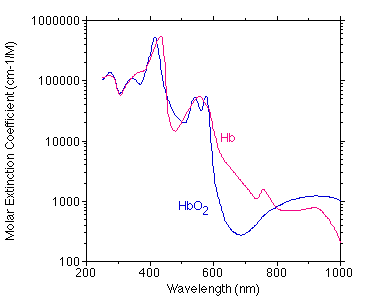Below is a comparison of Moaveni's data (points) with my compiled values (curve).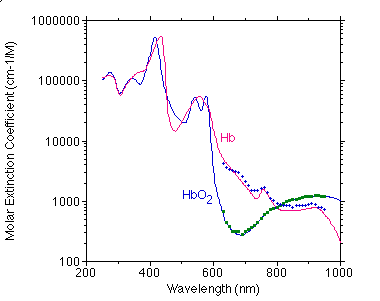Below is a comparison of Takatani's data (points) with my compiled values (curve).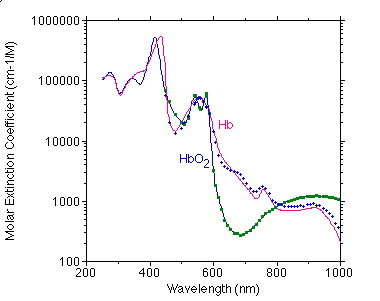#### Whole Blood

Hemoglobin has a normal concentration of 150g/liter of blood permits whole blood to carry 65 times more oxygen than does plasma at a PO2 of 100 mmHG. Hematocrit determines the fraction of the blood that is red blood cells. The red blood cells are primarily composed of hemoglobin (95% of the dry mass).

When arterial blood is 90% saturated, some of the hemoglobin molecules have four oxygens bound, some have three, and a few have tow or one. The statistical average of all oxygen bound to hemoglobin molecules relative to the total amount that can be bound is its oxygen staturation. One gram of O2 of functional hemoglobin combines with 1.34ml O2, the O2 capacity of normal blood is

(150g Hb/liter)(1.34ml O2 g Hb) = 200ml O2/liter.

But I just want a typical spectrum for blood in units I can understand...

Quit whining.

Assume 150 g Hb/liter. Then to convert the molar extinction coefficient e to an absorption coefficient, multiply by the molar concentration and 2.303,

μa(lambda) = (2.303) e(lambda) (150 g/liter)/(64,500 g Hb/mole)
= 0.0054e(lambda)

where μa is in (cm-1) and e(lambda) is the molar extinction coefficient for the wavelength of interest.